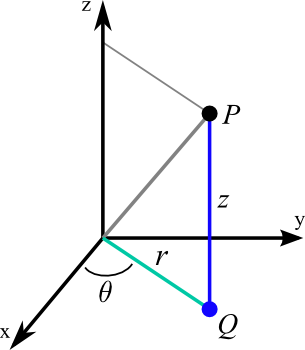# Math Insight

### Image: Cylindrical coordinatesIllustration of the definition of the cylindrical coordinates $r$, $\theta$, and $w$ of a point $P$. The coordinate $w$ is the height of $P$ above the $xy$-plane (equal to the $z$ of Cartesian coordinates). If the point $Q$ is the projection of $P$ to the $xy$-plane, then $\theta$ is the angle between the positive $x$-axis and the line from the origin to $Q$. The coordinate $r$ is the distance from $Q$ to the origin.

Image file: cylindrical_coordinates.png

Source image file: cylindrical_coordinates.svg
Source image type: Inkscape SVG